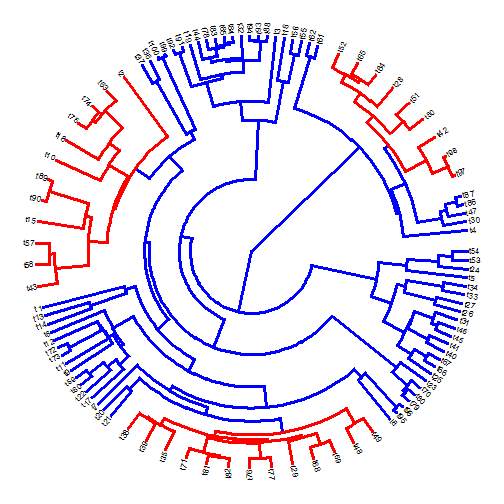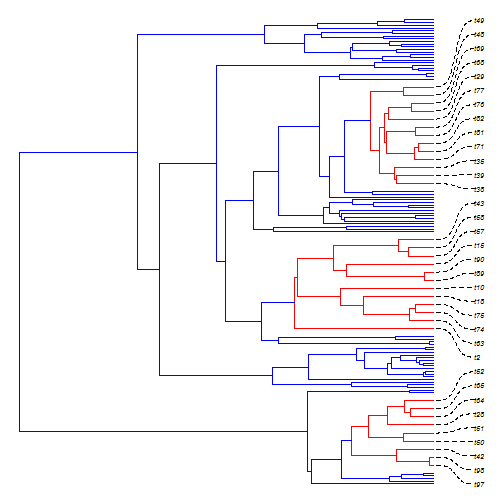## Sunday, June 4, 2017

### General function for expanding (or contracting) the tip-spacing of subtrees during visualization

A couple of days ago a phytools user asked me about using custom tip-spacing in a fan-style tree plot in R. I posted a fairly straightforward solution; however how it works is not super obvious, and it involves a lot of scripting, so I thought it might be worth adding this as a function in phytools.

The idea with the function is not to plot a tree with custom tip-spacing - we can already do that using `plotTree` or `plotSimmap`. Rather, the function reorders the tree (if it is not in “cladewise” order), computes the desired spacing of each specified subtree, and then returns an object consisting of the tree & a vector that we can use in our tree plot.

Here's the function:

``````expand.clade<-function(tree,node,factor=5){
cw<-reorder(tree)
tips<-setNames(rep(1,Ntip(tree)),cw\$tip.label)
get.tips<-function(node,tree){
dd<-getDescendants(tree,node)
tree\$tip.label[dd[dd<=Ntip(tree)]]
}
desc<-unlist(lapply(node,get.tips,tree=cw))
for(i in 2:Ntip(cw)){
tips[i]<-tips[i-1]+
if(names(tips)[i]%in%desc){
1
} else if(names(tips)[i-1]%in%desc){
1
} else 1/factor
}
obj<-list(tree=tree,tips=tips)
obj
}

## S3 method for the object class
cat("An object of class \"expand.clade\" consisting of:\n")
cat(paste("(1) A phylogenetic tree (x\$tree) with",Ntip(x\$tree),
"tips and\n   ",obj\$tree\$Nnode,"internal nodes.\n"))
cat("(2) A vector (x\$tips) containing the desired tip-spacing.\n\n")
}
``````

Here's an example:

``````tree
``````
``````##
## Phylogenetic tree with 100 tips and 99 internal nodes.
##
## Tip labels:
##  t4, t30, t47, t86, t87, t97, ...
##
## The tree includes a mapped, 2-state discrete character with states:
##  a, b
##
## Rooted; includes branch lengths.
``````
``````nodes<-c(108,140,168)
for(i in 1:length(nodes)) tree<-paintSubTree(tree,nodes[i],"b","a")
obj
``````
``````## An object of class "expand.clade" consisting of:
## (1) A phylogenetic tree (x\$tree) with 100 tips and
##     99 internal nodes.
## (2) A vector (x\$tips) containing the desired tip-spacing.
``````
``````plot(obj\$tree,colors=setNames(c("blue","red"),c("a","b")),
tips=obj\$tips,type="fan",fsize=0.7,lwd=3)
``````It also works for other tree styles. For instance:

``````obj<-expand.clade(tree,nodes,factor=3)
obj
``````
``````## An object of class "expand.clade" consisting of:
## (1) A phylogenetic tree (x\$tree) with 100 tips and
##     99 internal nodes.
## (2) A vector (x\$tips) containing the desired tip-spacing.
``````
``````plot(obj\$tree,colors=setNames(c("blue","red"),c("a","b")),
tips=obj\$tips,fsize=0.4,lwd=1,ftype="off",xlim=c(0,6.5))
tips<-unlist(lapply(nodes,foo,tree=tree))
nn<-sapply(tips,function(tip,tree) which(tree\$tip.label==tip),tree=tree)To get the final example to work, I had to push a fix to the function `linklabels`. The fix is already on GitHub & the functions of this post will be added soon.